DAY 9
1

Day9 - 理解 SVG viewport 與 viewboxviewport

viewbox ( min-x, min-y, width, height )

viewbox 對於第一次接觸的人來說，是很抽象的一個元素，第一因為不知道它是幹嘛用的，第二，就算你勉強了解了，還是不清楚該如何去設定。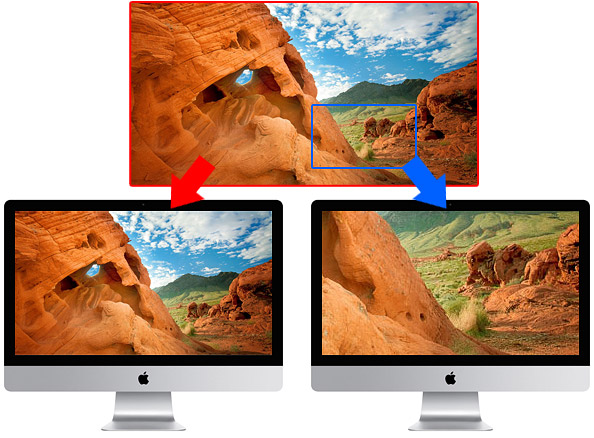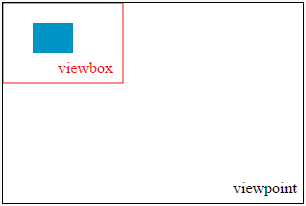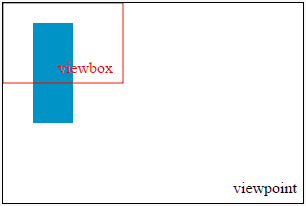> preserveAspectRatio ( align [meetOrSlice] )

preserveAspectRatio 是使用 viewbox 時，一個搭配服用的特別屬性，這個屬性必須要有 viewbox 才有作用，不然寫了就會直接被忽略掉，接著我們看到 preserveAspectRatio 裏頭具有兩個參數，第一個參數是必填的 align，第二個參數是選填的 meetOrSlice，也因此從字面上可以發現，preserveAspectRatio 是一個以 對齊為主，然後再選擇要自動填滿還是咖掉的屬性，了解之後，就讓我們來一一解釋。

1.align

align 參數是由兩個名詞所組成，這兩個名詞分別代表 viewbox 與 viewport 的 x 方向對齊模式，以及 y 方向的對齊模式，換句話說，可以想成：「水平置中 + 垂直靠上對齊」的這種感覺，不過在這個 align 的表現手法倒是很抽象，可以用下方的表格看出端倪：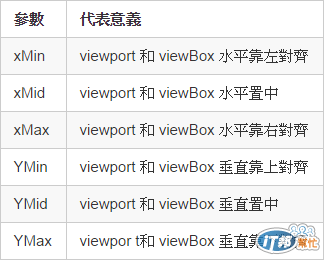<svg width="300" height="200" style="border:1px solid; background:#fff;" viewbox="0,0,100,160" preserveAspectRatio="xMinYMin slice">
<rect width="40" height="30" x="0" y="0" fill="#c00">
</rect>
<rect width="40" height="30" x="60" y="0" fill="#f80">
</rect>
<rect width="40" height="30" x="0" y="130" fill="#09c">
</rect>
<rect width="40" height="30" x="60" y="130" fill="#0c0">
</rect>
<rect width="40" height="30" x="30" y="65" fill="#000">
</rect>
</svg>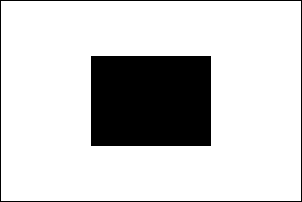<svg width="300" height="200" style="border:1px solid; background:#fff;" viewbox="0,0,100,160" preserveAspectRatio="xMidYMid slice">
<rect width="40" height="30" x="0" y="0" fill="#c00">
</rect>
<rect width="40" height="30" x="60" y="0" fill="#f80">
</rect>
<rect width="40" height="30" x="0" y="130" fill="#09c">
</rect>
<rect width="40" height="30" x="60" y="130" fill="#0c0">
</rect>
<rect width="40" height="30" x="30" y="65" fill="#000">
</rect>
</svg>

2.meetOrSlice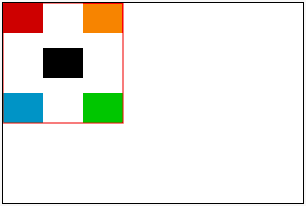<svg width="300" height="200" style="border:1px solid; background:#fff;" viewbox="0,0,120,120" preserveAspectRatio="xMinYMin meet">
<rect width="40" height="30" x="0" y="0" fill="#c00">
</rect>
<rect width="40" height="30" x="80" y="0" fill="#f80">
</rect>
<rect width="40" height="30" x="0" y="90" fill="#09c">
</rect>
<rect width="40" height="30" x="80" y="90" fill="#0c0">
</rect>
<rect width="40" height="30" x="40" y="45" fill="#000">
</rect>
</svg><svg width="300" height="200" style="border:1px solid; background:#fff;" viewbox="0,0,120,120" preserveAspectRatio="xMidYMid slice">
<rect width="40" height="30" x="0" y="0" fill="#c00">
</rect>
<rect width="40" height="30" x="80" y="0" fill="#f80">
</rect>
<rect width="40" height="30" x="0" y="90" fill="#09c">
</rect>
<rect width="40" height="30" x="80" y="90" fill="#0c0">
</rect>
<rect width="40" height="30" x="40" y="45" fill="#000">
</rect>
</svg>

SVG 30 天 ~ 就要醬玩31